# Acreage

Plot has a diamond shape, its side is 25.6 m long and the distance of the opposite sides is 22.2 meters. Calculate its acreage.

Correct result:

S =  568.32 m2

#### Solution: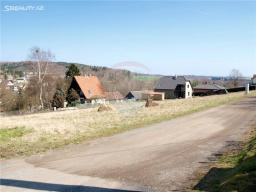We would be pleased if you find an error in the word problem, spelling mistakes, or inaccuracies and send it to us. Thank you!#### You need to know the following knowledge to solve this word math problem:

We encourage you to watch this tutorial video on this math problem:

## Next similar math problems:

• Here isHere is a data set (n=117) that has been sorted. 10.4 12.2 14.3 15.3 17.1 17.8 18 18.6 19.1 19.9 19.9 20.3 20.6 20.7 20.7 21.2 21.3 22 22.1 22.3 22.8 23 23 23.1 23.5 24.1 24.1 24.4 24.5 24.8 24.9 25.4 25.4 25.5 25.7 25.9 26 26.1 26.2 26.7 26.8 27.5 27.6 2
• Four prismsQuestion No. 1: The prism has the dimensions a = 2.5 cm, b = 100 mm, c = 12 cm. What is its volume? a) 3000 cm2 b) 300 cm2 c) 3000 cm3 d) 300 cm3 Question No.2: The base of the prism is a rhombus with a side length of 30 cm and a height of 27 cm. The heig
• KiteJohn a kite, which is diamond shaped. Its diagonals are 60 cm long and 90 cm long. Calculate: a) the diamond side b) how much paper John needs to make a kite if he needs paper on both sides and needs 5% of the paper for bending.
• DiamondRhombus has side 17 cm and and one of diagonal 22 cm long. Calculate its area.
• Dusana) Dusan break two same window, which has triangular shape with a length of 0.8 m and corresponding height 9.5 dm. Find how many dm2 of glass he needs to buy for glazing of these windows. b) Since the money to fix Dusan has not, must go to the paint job
• PrismCalculate the surface area and volume of a prism with a body height h = 10 cm and its base has shape of a rhomboid with sides a = 5.8 cm, b = 3 cm and the distance of its two longer sides is w = 2.4 cm.
• Acreage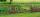What acreage has a rectangular plot whose diagonal is 34 meters long and one side has a length of 16 meters. ?
• Garden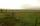The garden has two opposite parallel fences. Their distance is 33.1 m. Lengths in these two fences are 75.5 meters and 49.4 meters. Calculate the area of this garden.
• Diamond diagonalsCalculate the diamonds' diagonals lengths if the diamond area is 156 cm square and the side length is 13 cm.
• Cardboard boxWe want to make a cardboard box shaped quadrangular prism with rhombic base. Rhombus has a side of 5 cm and 8 cm one diagonal long. The height of the box to be 12 cm. The box will be open at the top. How many square centimeters cardboard we need, if we ca
• The farmer field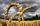The field has paralleogram shape with dimensions side a = 80 m and height 296 m. Two and two sides is at angle 80°. Calculate acreage of field in hectares.
• Rectangle A2dim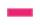Calculate the side of the rectangle, if you know that its area is of 2590 m2 and one side is 74 m.
• Rhombus 2Calculate the area of rhombus which has a height v=48 mm and shorter diagonal u = 60 mm long.
• Pine woodFrom a trunk of pine 6m long and 35 cm in diameter with a carved beam with a cross-section in the shape of a square so that the square had the greatest content area. Calculate the length of the sides of a square. Calculate the volume in cubic meters of lu
• Diamond perimeter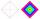Calculate the diamond circumference which area is 288 cm square and one diagonal has a size of 124 cm.
• PrismThe base of the prism is a rhombus with a side 30 cm and height 27 cm. The height of the prism is 180% longer than the side length of the rhombus. Calculate the volume of the prism.
• Pavilion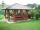The rectangular pavilion with dimensions 3.5 m and 2.75 m to be paved with square tiles of side 25 cm price of CZK 22 per 1 piece or rectangular tiles with sides of 20 cm and 15 cm in the price of CZK 11 per 1 pc. Which solution is cheaper (write its pric